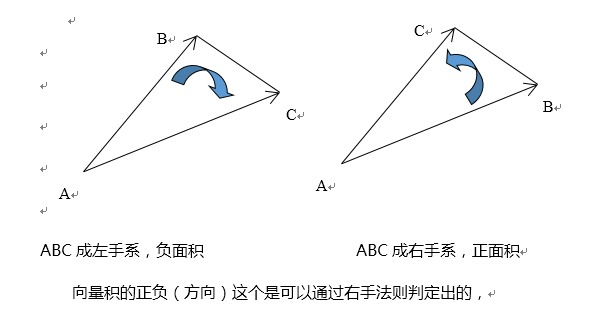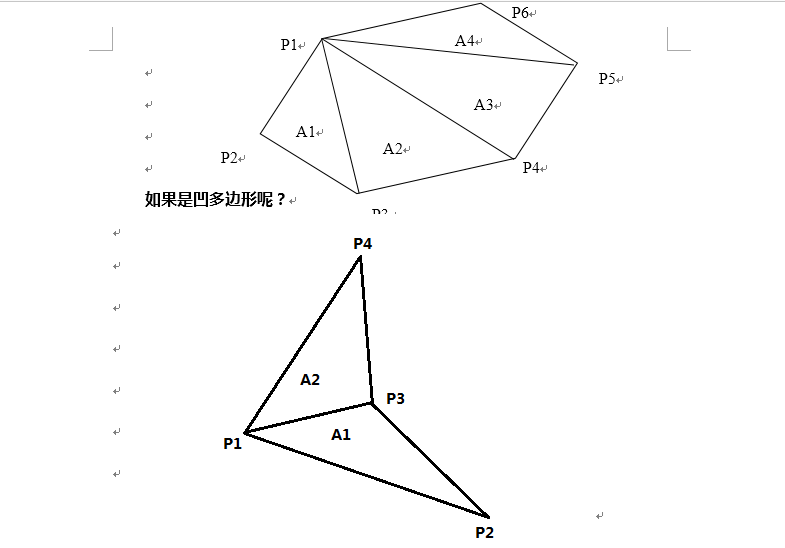# 【改革春风吹满地 HDU - 2036 】【计算几何-----利用叉积计算多边形的面积】

## 利用叉积计算多边形的面积【该图片来源：https://www.cnblogs.com/xiexinxinlove/p/3708147.html## AC代码

#include<iostream>
#include<cstdio>
#include<cstring>
#include<cmath>
#include<cstdlib>
using namespace std;
const int maxn = 100 + 10;
struct Point
{
int _x, _y;
Point operator-(Point b)
{
Point a;
a._x = _x - b._x;
a._y = _y - b._y;
return a;
}
};
int n;
Point p[maxn];
int Cross(Point a, Point b)
{
return a._x * b._y - a._y * b._x;
}
int Area(Point *p)
{
int ans = 0;
for(int i = 1; i < n - 1; i++)
ans += Cross(p[i]-p, p[i+1]-p);          //最好写成这样，清晰些，不容易出错
return ans;     //题目说的逆时针，故ans为正值，直接返回即可
}
int main()
{
//    freopen("input.txt", "r", stdin);
//    freopen("output.txt", "w", stdout);
while(cin >> n && n)
{
for(int i = 0; i < n; i++)
cin >> p[i]._x >> p[i]._y;
int area = Area(p);
printf("%.1lf\n", (double)area / 2.0);
}
}
posted @ 2019-08-24 17:36 AJudge 阅读(...) 评论(...) 编辑 收藏